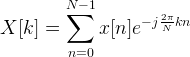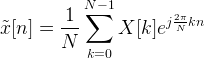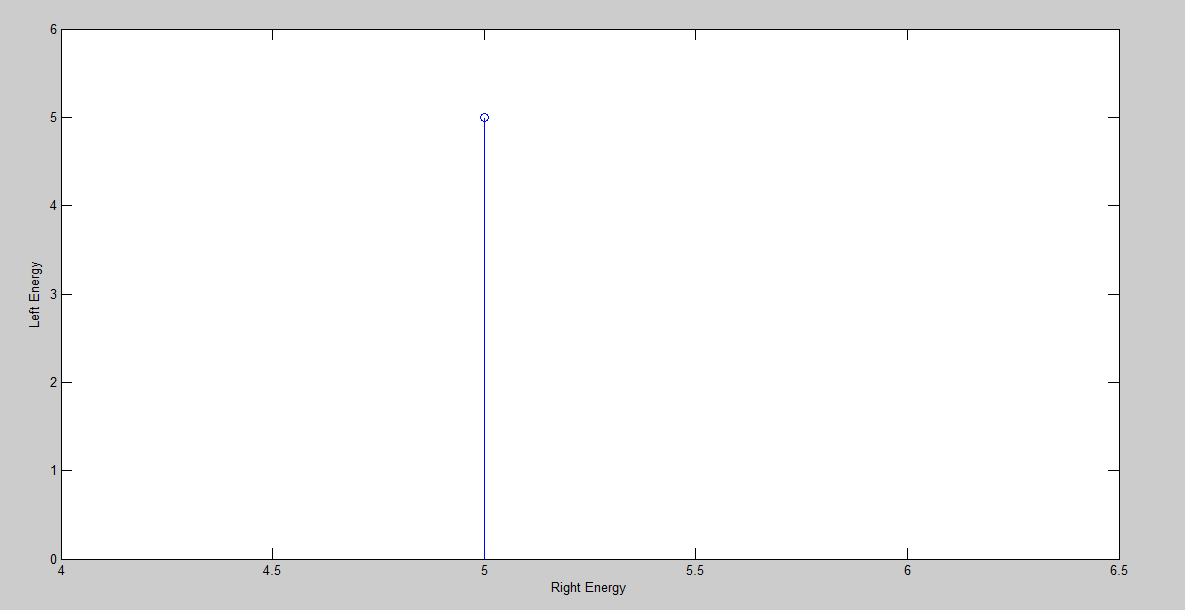# Parseval’s Theorem in MATLAB Compete (Code + Output)

In DSP(Digital Signal Processing), Parseval’s theorem usually refers to the result that the Fourier transform is unitary; loosely, that the sum (or integral) of the square of a function is equal to the sum (or integral) of the square of its transform.

For the DFT, Parseval’s relation is expressed: The left side of this equation is the total energy contained in the time domain signal, found by summing the energies of the N individual samples.

Suppose if the x[n] is a sequence of complex numbers of length N : xn={x0,x1,…,xN-1}, its N-point discrete Fourier transform (DFT)Xk={X0,X1,…,XN-1} is given byThe inverse discrete Fourier
transform is given byYou can perform Parseval’s Theorem with any Sinusoidal signal in MATLAB. To do Parseval’s theorem with any signal in Matlab, simply open and create a new Script (.m file) in Matlab.

Step 1:

Open MATLAB << Then on the top left corner click on the File option << Click New << Then click Script.

Step 2:

Now write the following Matlab code given below of Parseval’s therorem into the new script (.m file) and save the file in the Matlab directory with any name you want.

PARSEVAL’S THEOREM MATLAB CODE:

n = 1:10;

x = sin(n);

xmod = abs(x);

xmodsquared = xmod.^2;

energy_left = sum(xmodsquared)

N = length(x);

f = fft(x,N);

fmod = abs(f);

fmodsquared = fmod.^2;

energy_right = sum(fmodsquared)/N

stem(energy_right,energy_left)

xlabel(‘Right Energy’)

ylabel(‘Left Energy’)

Step 3:

Now Run the code, after you have saved the file.

OUTPUT:

energy_left =

5.0014

energy_right =

5.0014

FIGURE:This is how you can perform Parseval’s Theorem in MATLAB. Hope you like this article. If you want to learn more about MATLAB you can read and view all the blog posts related to MALTAB here. MATLAB Learning

If you need any help and assistance, please comment down below so that we can help you. Good Luck!!

See More…